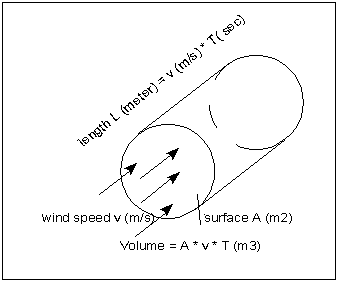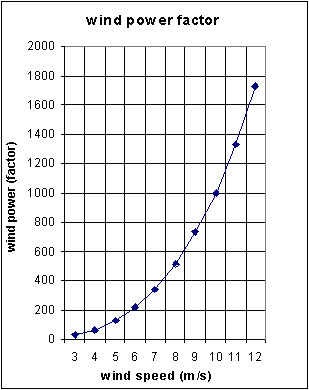The truth about wind speed & wind power measurements

by PETER H. van EMDEN

#### The calculation of wind power from wind speed data:

Remember from the lessons in basic physics:
the energy of a moving particle with mass m [kg] and speed v [m/s] is:

Energy = 1/2 * m * v2     (this is called the kinetic energy, or "energy of movement").Wind is moving air, which has a mass and a speed (and a direction). The mass of wind can be calculated when we realize that during T seconds a volume of air has passed the surface A (e.g. the surface of a wind turbine), which is equal to

A * L = A * v * T (m3)
(v = wind speed in m/s, T is time in seconds)
Fig. 1.

When r is the density of air (kg/m3) the passed mass during T (seconds) is:
Mass m [kg] = A * v * T * r
so the kinetic energy of the wind during T seconds is:

Energy [Joule] = 1/2 * m * v2 = 1/2 * A * v * T * r * v2 = 1/2 * A * T * r * v3

As power [Watts] is Energy [Joule] per second, the available wind power is equal to: (divide by T seconds)

Wind power [Watts] = 1/2 * A * v * r * v2 = 1/2 * A * r * v3

So wind power is proportional to the cube of the wind speed! A small deviation in measured windspeed result in a big deviation in calculated wind power, refer fig. 2 and fig. 3. We call v3 the wind power factor, see fig. 2:Fig. 2.

Note that r depends on the atmospheric pressure and temperature:
proportionial to atmospheric pressure(hP) / Temperature (Kelvin).
So for wind power measurements the pressure and temperature should also be measured!
Depending on the quality and efficiency of a wind turbine the wind power (power in the wind) can be converted into mechanical or electrical energy.Fig. 3.

For windpower feasibility (or evaluation) studies it is important to determine the potential wind power, before high investments are made. In order to obtain an accurate calculation of the wind power (within appr. 10 %), the wind speed should be measured accurately, so with a small deviation!
As above 4 m/s a wind turbine is producing electric energy an acccuracy between 0,2 m/s and 0,1 m/s is recommended! Note that the resolution (smallest "step" in which a value is expressed) of the wind measurement should be small compared to the required accuracy (e.g resolution 0,05 m/s when accuracy is 0,2 m/s).

Above information is important during selection of wind monitoring equipment.
EKOPOWER supplies wind monitoring equipment (EKO21B) with standard accuracy of 0,2 m/s;
with optional individual MEASNET*)calibration certificate of even 0,1 m/s, so high accuracy is guaranteed! This is required when high investments are planned or for technical-scientific measurements. Also the air-temperature and atmospheric pressure should be measured! For the calculation of the energy production of a wind turbine is required:

• accurate wind speed data: wind speed frequency distribution, wind rose, turbulence intensity, Weibull parameters
• the characterictic of the planned wind turbine (power curve)

EKOPOWER supplies all the required hardware and software tools to carry out such calculations.
It is also important to carry out the measurements according to the international standards, like IEC 61400-12 and WMO, AWEA, IEA etc.

*) MEASNET: the new European accuracy standard for wind measurements